#### Energy Changes in Chemical-Physical Processes, Chemistry tutorial

Introduction:

Energy is the capability or capacity to do work. Energy appears in various forms, some of which are kinetic, potential, heat, chemical, electrical and light. The conversion of energy from one form to the other takes place all the time in natural and man-made activities. Some of the illustrations of energy conversions are:

i) Food (that is, a form of chemical energy) is transformed to heat whenever digested.

ii) Fuel (that is, petrol or diesel) as well a form of chemical energy is transformed to heat whenever it burns and then to mechanical energy for motion.

iii) A stone dropped from a height holds potential energy that is transformed steadily to kinetic as it falls and lastly to heat whenever it strikes the ground. In all energy conversions, energy is conserved. The law of conversion of energy defines that energy is neither created nor destroyed in its transformation.

Each and every matter consists of its own characteristic energy whenever energy is transformed from one form to the other, whether in a chemical or physical process, an energy will accompany the procedure.

Energy Changes in Chemical Processes:

Heat content and heat of reaction:

The energy of a chemical substance holds as an outcome of its structure and physical state is known as its heat content or enthalpy. The change in energy which accompanies a chemical reaction is known as the heat of reaction. In energy computation, enthalpy and enthalpy change are represented by the symbols H and DH correspondingly.

The absolute value of the heat content can't be evaluated for a chemical substance however the heat or enthalpy change can be evaluated or calculated.

For a chemical reaction,

DH reaction = HP - HR here Hp and HR are the enthalpies of the product and reactant correspondingly. For reactions comprising numerous reactants and products the equation becomes

ΔHreaction = ΣHP = ΣHR where Σ signifies summation.

Exothermic and endothermic reactions:

A chemical reaction is either goes along by evolution or absorption of heat. Proof of heat evolution is a raise in temperature. The reaction container is warm or hot to the hand. This is the exothermic reaction. For endothermic reaction, the temperature reduces and the reaction container feels cold to the hand due to heat absorption from the surroundings.

Remember the equations:

ΔHreaction = HP - HR and

ΔHreaction = ΣHP - ΣHR

If the total heat content of the products is less than that of the reactants the enthalpy change (ΔH) is less than zero that is, (ΔH) is negative. The difference in the heat contents is given off to the surrounding. The exothermic reaction is a reaction in which heat is given off and ΔH is negative. For the endothermic reaction ΔH is positive that is, Hp > HR and heat is absorbed from the surroundings. The endothermic reaction is a reaction in which the heat is absorbed and ΔH is positive.

Energy level diagrams:

The energy change in a physical or chemical method can be symbolized on a plot of potential energy change against the time of reaction. This kind of diagram is termed as an energy level diagram.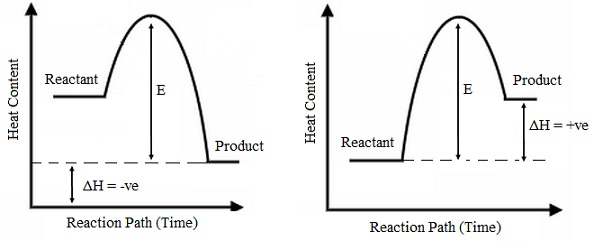Fig: Exothermic and Endothermic reaction

The energy change represented by 'E' on the diagrams is termed as the activation energy. The activation energy is the minimum energy required by the reactant before reaction can take place. All reactions whether endothermic or exothermic need the activation energy.

Whenever reactions occur between the molecules or atoms, it generally comprises the breaking of old bonds among the atoms or molecules and the formation of new ones. Such methods are not consecutive however take place concurrently, at which point an activated complex is stated to be formed. The activated complex is a temporary stage of the reactants whose time period is extremely short.

The standard state:

Enthalpy and enthalpy change based on the temperature and pressure of the reaction. The reference point of enthalpy and enthalpy computations is 25°C (298K) and 760 mm Hg pressure (that is, 1 atmosphere) for gas reactions and a concentration of 1.0 mol dm-3 for reactions in solution. The heat change evaluated beneath the conditions stated above is termed as the standard heat (or enthalpy) change. This is represented by the symbol ΔH°. The enthalpy at standard state is represented by the symbol H°. The standard enthalpy change of a reaction is the amount of heat evolved or absorbed whenever a chemical reaction takes place between the molar quantities of the reactant as symbolized in the equation of the reaction in standard conditions. This is represented by the symbol ΔHoreaction.

Standard enthalpy of a compound is the heat content at standard state. The standard enthalpy of pure elements in their normal state is zero. The latter statement means that for H2(g), Na(s), C(s) and so on Ho is 0.

Thermochemical equations:

The heat contents of reactants and products of a reaction based not only on their chemical structures however as well on their physical states. We are familiar that the temperature and pressure influence the enthalpy and enthalpy change of reactions; therefore the standard state is stated as a reference point. The given is a list of factors which can influence the enthalpy of a compound.

1) Amount of reactants and products: The heat of a reaction is proportional to the quantity or amount of reactants used up or products made. The enthalpy change (ΔH) is generally represented per mole of a reactant or a product.

2) A change of temperature or pressure for gases: It is thus extremely obligatory to define the pressure and temperature of measurement if different from the standard state.

3) The physical state of the reactants and products: This is significant to define the physical state of the reactants and products. The physical state is pointed as subscript after the chemical symbols of reactants and products as (g) for gas (l) for liquid and (s) for solid. For a salt or ion in solution the symbol (aq) is employed.

4) The concentration of salt or ions in solution: Due to the stated factors that can influence the enthalpy of compounds, it is requisite that a chemical reaction should be symbolized correctly in energy computations.

The chemical reaction equation that provides the physical states of the reactants and products and also the heat change which accompanies the reaction is termed as the thermo-chemical equation for the reaction

Illustration: C(s) + O2(g) → CO2                 ΔH = - 393 kJmol-1

H2(g) + 1/2 O2(g) → H2O(l)       ΔH = - 285 kJmol-1

Nacl(aq) → Na+(aq) + Cl-          ΔH = - 13.6 kJmol-1

Types of Heat of Reaction:

Reactions are of numerous types. The heat change will be stated and named accordingly for formation, combustion, neutralization and solution. The standard heat is stated in each case.

Standard heat (enthalpy) of formation ΔHof:

The standard enthalpy of formation of a compound is the heat evolved or absorbed whenever one mole of the compound is made from its elements in their normal state under standard conditions.

H2(g) + 1/2 O2(g) → H2O(l)

H2(g) + 1/2 O2(g) → H2O(g)

For a particular chemical compound there is just one chemical equation which fits the above definition of heat of formation. Now write the chemical equations for the formation of CO(g) and CaCO3(g)

Heat of formation is helpful in the computation of heat of reaction.

ΔHoreaction = ΣΔHof of products - ΣΔHof of reactants

Standard heat (enthalpy) of combustion ΔH°C:

It is the heat evolved if 1 mole of compound is fully burnt in oxygen beneath standard conditions. It will be noted that combustion reactions are exothermic reactions. Combustion reactions are extremely significant as they form the major source of energy for industrial and domestic aims. Even the food we eat supply us energy. It is burnt off all through digestion. Heat of combustion is helpful in the computation of heat of formation and reaction

ΔHof = ΣΔHoc of reactants → ΣΔHoc of products

Standard heat (enthalpy) of Neutralization, ΔHoneu:

This is the quantity of heat evolved whenever 1 mole of H+ from an acid reacts by 1 mole OH from an alkali to make a mole of water beneath standard conditions.

ΔHof = ΣΔHoneu of reactants → ΣHoneu Products

Therefore, ΔHoreaction = ΣΔHoneu of reactants → ΣΔHoneu of products

Neutralization is the reaction of an acid having an alkali to provide salt and water only. Strong acids and bases are strong electrolytes. They ionize fully in solution. For these acids and bases, neutralization is the reaction of H+ and OH- to provide water

H+(aq) + OH-(aq) → H2O(l)

This is the reason, why the heat of neutralization is fundamentally constant for strong acids and bases.

For illustration:

HNO3 (aq) + KOH (aq) → KNO3 (aq) + H2O (l)

ΔH = - 57.3 KJmol-1

HCl (aq) + NaOH (aq) → NaCl (aq) + H2O (l)

ΔH = - 57.1 KJmol-1

Weak acids and bases are weak electrolytes and are just partially dissociated in solution. Part of the energy of neutralization is expended to effect complete dissociation. This is the reason, why the heat of neutralization for weak acid or weak base reaction is lower than for the strong acid and base combination.

Example:  NH4OH (aq) + HCl (aq) → NH4Cl (aq) + H2O (l)

ΔH = - 51.5 KJ mol-1

Standard heat (enthalpy) of solution ΔHosoln:

Dissolution is the physical method in which a solute mixes homogeneously by a solvent. Example: sugar solution or brine (that is, solution of common salt in water).

The separation to solute and solvent is through physical methods. For the recovery of solute, evaporation is employed and for solvent recovery simple distillation is employed.

For the ionic solid dissolving in water, a two step method takes place.

1) Lattice breaking that resultant in the formation of Na+ (g) and Cl-(g). This method is an endothermic process. The energy needed is the lattice energy (ΔHo lattice) ΔH is positive.

2) The free ions are attracted to the water, molecules and they become hydrated. This method discharges energy. This is exothermic and ΔH negative.

Such two steps illustrate the dissolution method and the resultant heat of solution based on the relative magnitude of the lattice energy and hydration energy.

The method is symbolized as in the equations.

NaCl (s) → Na+ (g) + Cl- (g)

↓

Na+ (aq) + Cl- (aq)

ΔHsoln = ΔHlattice + ΔHhydration

The dissolution method will discharge heat energy (that is exothermic) if ΔHhydration > ΔHlattice or else the dissolution method will be the endothermic process.

The standard heat of solution is the heat (that is, enthalpy) evolved or absorbed whenever one mole of a substance is dissolved in so much water that dilution outcomes in no change in heat. For a mole of the solid as stated in the definition, the lattice energy ΔH lattice is a constant however the hydration energy raises with dilution. Diluting a concentrated solution will thus lead to further heat change known as the heat of solution. At very high dilution whenever the ions have been fully hydrated, further dilution will not lead to heat change as both ΔHlattice and ΔHhydration are constant.

Enthalpy of change of state:

There are three major physical transformations that can take place for a given substance. These are the solid → liquid, liquid → gas and solid → gas known as melting, vaporization and sublimation correspondingly. The molar heat of fusion (ΔHfusion) s the heat energy needed to transform a mole of the solid to the liquid at its melting point. The molar heat of vaporization (ΔHvap) is the heat energy needed to transform a mole of the liquid to the vapor at boiling point. The molar heat of sublimation ΔHsub is the heat energy needed to transform a mole of the solid to gas at similar temperature.

These heats are symbolized as illustrated: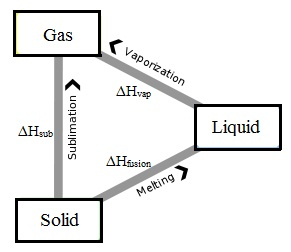Fig: Enthalpy of change of state

Determination of the Heat of Reaction:

The measurement of absolute enthalpies of compounds is not possible however enthalpy changes can be evaluated in the laboratory. The measurement of heat changes which accompany reactions is known as the Calorimetry and Calorimetry experiments are completed in specially insulated containers termed as calorimeters.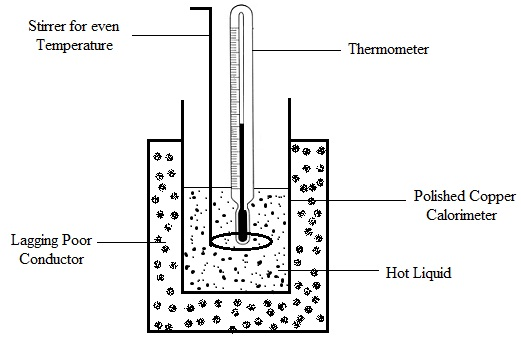Fig: Calorimeters

The term Calorimetry and calorimeter come from the word calorie. A calorie is the amount of heat energy needed to increase the temperature of one gram of water through 1o centigrade. The SI unit of heat is joules (J) and one calorie is equal to 4.2 joules. The calorimeters are of many kinds. The bomb calorimeter is a somewhat sensitive calorimeter employed in the measurement of heat of combustion.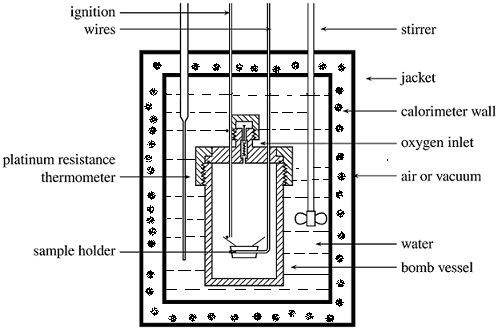Fig: A bomb calorimeter

Simple and less sensitive calorimeters can be adapted for the measurement of small heat changes like in neutralization reactions and solutions. Such adapted calorimeters should be well insulated and measurement should be as fast as possible to decrease errors. The figure below represents an adapted calorimeter for the determination of heat of neutralization.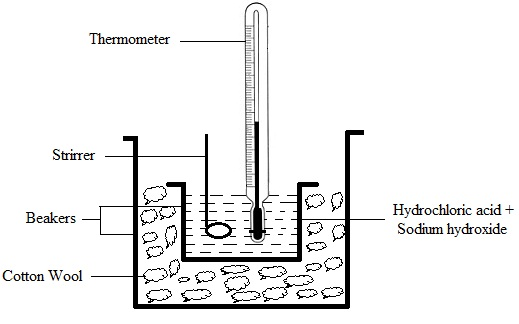Fig: Determination of the heat of neutralization

In all the Calorimetry experiments, the main measurement is the temperature change which accompanies the reaction of a known amount of the reactants in a calorimeter of acknowledged heat capacity (that is, the Heat capacity is the heat energy required to increase the temperature via 1°C). The heat change (that is, enthalpy) is computed by equating the heat energy gained via the calorimeter and its contents to the heat given off or absorbed in the reaction. Most of the Calorimetry experiments are done at temperature and pressures dissimilar from the standard state.

The heat of numerous reactions can't be measured directly due to experimental difficulties. The nature of the reactants, that is, the rate of reaction and the magnitude of the heat are a few such difficulties example: some reactions are explosive in nature because of the huge amount of heat which accompanies them while a few are too slow for any accurate measurements to be possible.

Na (s) + H2O (l) → NaOH (aq) + H2 (g)

This reaction is explosive due to the huge heat of reaction. Where the heats can't be evaluated, they can be computed from the heats of formation or the heats of combustion of the reactants and products.

Remember,

ΔHReaction = ΣΔHf product - ΣΔHf reactant

Or ΔHReaction = ΣΔHc of reactants - ΣΔHc of products

Tutorsglobe: A way to secure high grade in your curriculum (Online Tutoring)